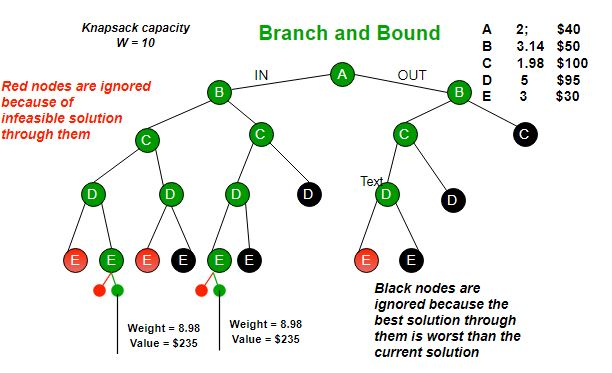# Branch and Bound Algorithm

• Last Updated : 10 Aug, 2022

## What is Branch and Bound Algorithm?

Branch and bound is an algorithm design paradigm which is generally used for solving combinatorial optimization problems. These problems are typically exponential in terms of time complexity and may require exploring all possible permutations in worst case. The Branch and Bound Algorithm technique solves these problems relatively quickly.

## Example to show working of Branch and Bound Algorithm

Let us consider the 0/1 Knapsack problem to understand Branch and Bound.

There are many algorithms by which the knapsack problem can be solved:

Let’s see the Branch and Bound Approach to solve the 0/1 Knapsack problem: The Backtracking Solution can be optimized if we know a bound on best possible solution subtree rooted with every node. If the best in subtree is worse than current best, we can simply ignore this node and its subtrees. So we compute bound (best solution) for every node and compare the bound with current best solution before exploring the node.

Example bounds used in below diagram are, A down can give \$315, B down can \$275, C down can \$225, D down can \$125 and E down can \$30.If you like GeeksforGeeks and would like to contribute, you can also write an article and mail your article to review-team@geeksforgeeks.org. See your article appearing on the GeeksforGeeks main page and help other Geeks.

Please write comments if you find anything incorrect, or if you want to share more information about the topic discussed above

My Personal Notes arrow_drop_up

Writing code in comment? Please use ide.geeksforgeeks.org, generate link and share the link here.